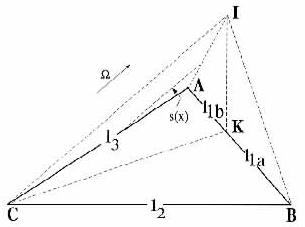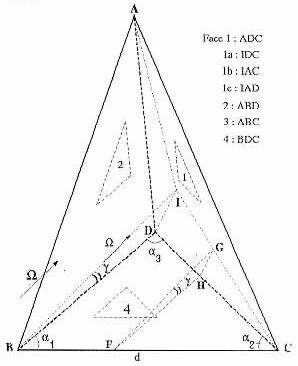### SESSION 5 DISCRETE ORDINATES METHOD

Chairman: B. Webb

ASSESSMENT OF DISCRETE ORDINATES METHOD FOR RADIATIVE TRANSFER IN CYLINDRICAL FURNACES

Nevin Selçuk* and Nuray Kayakol**
*Department of Chemical Engineering
Middle East Technical University
Ankara 06531, Türkiye
**Glass Research Center
Sisecam, Istanbul, Türkiye

The solution accuracy of Sn or Discrete Ordinates Method (DOM) was assessed by comparing its predictions with exact solutions reported earlier on a cylindrical enclosure problem with steep temperature gradients. The enclosure has interior black walls and an absorbing-emitting medium of constant properties. Predictive accuracy was investigated for two angular quadrature schemes: 1) the Sn discrete ordinates approximation (S2 and S4) and 2) the modified piecewise constant angular (PCA) approximation. Comparative testing shows that all predictions agree well with the exact solutions and that Sn approximations produce slightly better accuracy than the modified PCA approximation in radiative heat flux densities. The sensitivity of the predictions of the DOM to radiative properties was also examined by performing calculations for various absorption coefficients in the range 0.2 to 2.0. Comparative testing shows that the discrepancies between the predictions increase with the coefficient. The sensitivity analysis performed illustrates that testing a radiation model by predicting wall heat fluxes from measured gas and wall temperatures and estimated radiative properties and comparing the predictions with the measurements may be misleading. Hence, the accuracy of a radiation model should be tested in isolation from radiative property estimation.

Oscillations in the predicted dimensionless flux densities are present for low values of gas absorption coefficient but get smaller as the coefficient increases.

RADIATIVE EQUILIBRIUM OF AN HYDROGEN-HELIUM MIXTURE WITHIN AN ELLIPSOIDAL SHELL USING THE DISCRETE ORDINATES METHOD AND A SPECIFIC FREQUENCY INTEGRATION NIETHOD

R. VAILLON*, M. LALLEMAND*, D. LEMONNIER* and C. STEHLE**
* LET, UMR CNRS 6608, ENSMA, 86960 Futurosco e FRANCE
** DARC, UPR CNRS 176, Observatoire de Paris, 92195 Meudon Cedex, FRANCE

ABSTRACT- The radiative equilibrium of an hydrogen-helium ellipsoidal gas layer confined between an internal black surface at prescribed temperature and an external one either transparent or black is investigated. The temperature field is calculated by considering the coupling between the chemistry and the optical properties of the reactive medium in the range [3000 - 30000] K. The theoretical determination of the hydrogen-helium mixture absorption properties is performed under the assumption of local chemical and thermodynamical equilibria in the working temperature range, at a pressure of one atmosphere and a given initial molar fractions of the mixture. Absorption cross-sections calculations are presented for the following processes: bound-free and free-free electronic transitions of the negative hydrogen ion, bound-free, free-free and bound-bound transitions of the hydrogen atom. The determination of the lines shapes is carried out by means of the Model Microfield Method. Since total radiative quantities have to be calculated, a specific frequency integration method suitable for the hydrogen-helium absorption coefficient spectrum is presented. It is based on the constitution of a spectral data bank that makes total radiative quantities integration fast and accurate. Finally, the proposed method is used to solve the radiative equilibrium of such a system. The spectral directional intensity and incident energy fields are determined by using the Discrete Ordinates Method extended to orthogonal curvilinear coordinates.

ANALYSIS OF RADIATIVE HEAT TRANSFER IN ENCLOSURES OF COMPLEX GEOMETRY USING THE DISCRETE ORDINATES METHOD

A. CHARETTE*, M. SAKAMI* and V. LE DEZ**
* Groupe de Recherche en Ingénierie des Procédés et Systèmes, Département des
Sciences Appliquées, Université du Québec à Chicoutimi, 555, boulevard de 1'université,
Chicoutimi, G7H 2B 1, Québec, Canada
** Laboratoire d'Etudes Thermiques, E.N.S.M.A
(URA 1403) B.P 109
86960 Futuroscope, France

This paper presents a new discrete ordinates algorithm for the numerical treatment of radiative participating media in both two and three-dimensional enclosures. The algorithm is based on the utilization of general characteristic relations in lieu of the traditional differencing schemes for the spatial marching procedure. It is ideally suited for the treatment of complex geometries, the grid being formed from triangles (2D) or tetrahedra (3D). The method uses the techniques for recognition of neighboring cells and is exempt of any numerical oscillation. The paper summarizes the technique by presenting a unified derivation for 2D and 3D cases.

According to the discrete ordinates method (DOM), the radiative transfer equation (RTE) may be written for each discrete direction in cell i as:(1)

whereanddefines a quadrature of M discrete directionsto which are associated the weights wm. Di stands for the cell domain (area of a triangle or volume of a tetrahedron), bki for the boundaries (length of a segment or area of a triangle). Imi and Ikmi are averaged intensities in the cell domain and cell boundary, respectively.

To close the set of equations, lateral intensities Ikmi are needed. These are found by performing the integration of the non-conservative form of the RTE over an optical path t = sf - si in a given cell. The procedure leads to the following exponential scheme:(2)

where:(3)

Imsi is the incoming intensity at a given point on a cell and Imsf is the outgoing intensity of the ray after it travelled through the cell.

The new method consists in integrating the exponential scheme over the complete domain of a cell. Fig. 1 illustrates the technique used for 2D and 3D cases.

In the two-dimensional case (Fig. la), it can be shown that when one segment receives radiation from the two other segments, the average intensity on segment 1 may be expressed as:(4)Fig. la. Case where one face of a triangle (2D cell) receives radiation from the two other faces.Fig. lb. Case where one face of a tetrahedron receives radiation from the three other faces.

wherebeing the maximum optical thickness associated with the impact on the plane normal to ABC and containing AB.

Similar equations can be obtained for the 3D case. For the special situation where one face receives radiation from the three other faces (tetrahedron in Fig. lb), one obtains:(5)

whereis the maximum optical thickness associated with the impact on subface IDC.

Other situations (different radiation directions relative to the orientation of the cell) can be encountered. However the procedure is similar.

The new algorithm has been tested for a number of geometries and conditions, and the results compare favorably with those obtained by other authors. We report here a case that was not published earlier and shows the flexibility of our method. The chosen enclosure, depicted in Fig.2, is delimited by a parallelepiped and an hemisphere. The medium is emitting-absorbing and its temperature is 1000 K. The walls are black at 500 K. Solutions were obtained using 10220 tetrahedra arranged in an increasingly unstructured way as the hemisphere is approached, with 72 triangles on each face of the parallelepiped. The predicted S4 solution of the radiative heat flux in the z direction along a line at x = 1.17m and y = 0 for different values of the absorption coefficient is shown in Fig. 3. The fluxes are compared to those obtained in the absence of the hemisphere. For high absorption coefficients, the effect of the hemisphere obstruction becomes less significant and the flux distribution tends to be comparable to that obtained with the parallelepiped alone. For high values of z (far from the obstruction) the fluxes are identical to those of the parallelepiped (for all values of k). Conversely, when z is low, the effect of the obstruction is clearly perceptible.Fig. 2. Complex enclosure bounded by a cubical cavity and an hemispherical obstruction.Fig. 3. Net wall heat fiux along a vertical line of the parallelepiped / hemisphere cavity defined by x = 1.17m, y = 0; effect of the presence of the hemisphere and of the absorption coefficient (w = 0, e = 1.0)

A new DOM algorithm for radiative participating media has been developed. This technique differs from the classical one by replacing the traditional differencing schemes with characteristic equations issued from the formal integration of the RTE. A general formulation allows it to handle either 2D or 3D situations. Furthermore, since the grid is formed from triangular or tetrahedral cells that can be arranged in any unstructured manner, the algorithm can be easily adapted to arbitrary geometries. The method is unconditionally stable and does not generate any numerical oscillation. In this paper, after assessing the accuracy of the new algorithm against results taken from the literature for cases of regular geometry, we prove its flexibility by applying it to two non- conventional enclosures: an infinite half elliptical ring (2D) and a parallelepiped containing an hemispherical obstacle (3D).